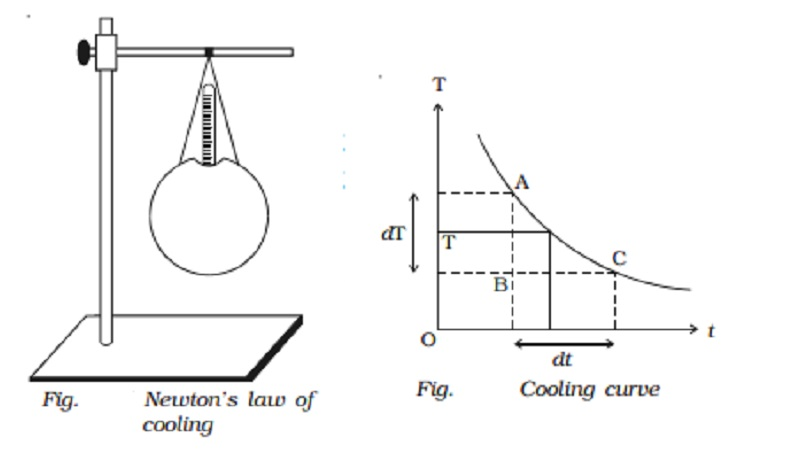Home | | Physics | | Physics | Experimental verification of Newton's law of cooling

# Experimental verification of Newton's law of coolingNewton?s law of cooling states that the rate of cooling of a body is directly proportional to the temperature difference between the body and the surroundings.

Newton s law of cooling

Newton s law of cooling states that the rate of cooling of a body is directly proportional to the temperature difference between the body and the surroundings.

The law holds good only for a small difference of temperature. Loss of heat by radiation depends on the nature of the surface and the area of the exposed surface.

Experimental verification of Newton s law of cooling

Let us consider a spherical calorimeter of mass m whose outer surface is blackened. It is filled with hot water of mass  m1.    The    calorimeter  with a thermometer is  suspended  from  a  stand (Fig.).The calorimeter and the hot water radiate heat energy to the surroundings. Using a stop clock, the temperature is noted for every 30 seconds interval of time till the temperature falls by about 20o C. The readings are entered in a tabular column.

If the temperature of the calorimeter and the water falls from T1 to T2 in t seconds, the quantity of heat energy lost by radiation

Q = (ms + m1s1) (T1  ? T2), where s is the specific heat capacity of the material of the calorimeter and s1 is the specific heat capacity of water.

Rate of cooling = Heat energy lost / time taken

Q / t = (ms + m1s1 )(T1 ? T2 )  /  t

If the room temperature is To, the average excess temperature of the calorimeter over that of the surroundings is  ( [ (T1 + T2) / 2 ] - T0)

According to Newton?s Law of cooling, Q/t α ( [ (T1 + T2) / 2 ] - T0)

(ms + m1s1 )(T1 ? T2 )   /  t[((T1+T2)/2) ? T0]  = constant

The time for every 4o fall in temperature is noted. The last column in the tabular column is found to be the same. This proves Newton?s Law of cooling.

Table Index : Newton?s law of cooling

1.     Temperature range

2.     Time t for every 4o fall of temperature

3.     Average excess of temperature   ( [ (T1 + T2) / 2 ] - T0)

4.     ( [ (T1 + T2) / 2 ] - T0)tA cooling curve is drawn by taking time along X-axis and temperature along Y-axis (Fig.).From the cooling curve, the rate of fall of temperature at T is dT/dt = AB/BC

The rate of cooling dT/dt is found to be directly proportional to (T - To). Hence Newton?s law of cooling is verified.

Study Material, Lecturing Notes, Assignment, Reference, Wiki description explanation, brief detail

Related Topics